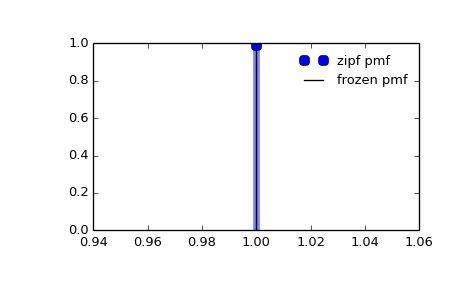# scipy.stats.zipf¶

scipy.stats.zipf = <scipy.stats._discrete_distns.zipf_gen object at 0x450c064c>[source]

A Zipf discrete random variable.

As an instance of the rv_discrete class, zipf object inherits from it a collection of generic methods (see below for the full list), and completes them with details specific for this particular distribution.

Notes

The probability mass function for zipf is:

zipf.pmf(k, a) = 1/(zeta(a) * k**a)


for k >= 1.

zipf takes a as shape parameter.

The probability mass function above is defined in the “standardized” form. To shift distribution use the loc parameter. Specifically, zipf.pmf(k, a, loc) is identically equivalent to zipf.pmf(k - loc, a).

Examples

>>> from scipy.stats import zipf
>>> import matplotlib.pyplot as plt
>>> fig, ax = plt.subplots(1, 1)


Calculate a few first moments:

>>> a = 6.5
>>> mean, var, skew, kurt = zipf.stats(a, moments='mvsk')


Display the probability mass function (pmf):

>>> x = np.arange(zipf.ppf(0.01, a),
...               zipf.ppf(0.99, a))
>>> ax.plot(x, zipf.pmf(x, a), 'bo', ms=8, label='zipf pmf')
>>> ax.vlines(x, 0, zipf.pmf(x, a), colors='b', lw=5, alpha=0.5)


Alternatively, the distribution object can be called (as a function) to fix the shape and location. This returns a “frozen” RV object holding the given parameters fixed.

Freeze the distribution and display the frozen pmf:

>>> rv = zipf(a)
>>> ax.vlines(x, 0, rv.pmf(x), colors='k', linestyles='-', lw=1,
...         label='frozen pmf')
>>> ax.legend(loc='best', frameon=False)
>>> plt.show()Check accuracy of cdf and ppf:

>>> prob = zipf.cdf(x, a)
>>> np.allclose(x, zipf.ppf(prob, a))
True


Generate random numbers:

>>> r = zipf.rvs(a, size=1000)


Methods

 rvs(a, loc=0, size=1, random_state=None) Random variates. pmf(x, a, loc=0) Probability mass function. logpmf(x, a, loc=0) Log of the probability mass function. cdf(x, a, loc=0) Cumulative density function. logcdf(x, a, loc=0) Log of the cumulative density function. sf(x, a, loc=0) Survival function (1 - cdf — sometimes more accurate). logsf(x, a, loc=0) Log of the survival function. ppf(q, a, loc=0) Percent point function (inverse of cdf — percentiles). isf(q, a, loc=0) Inverse survival function (inverse of sf). stats(a, loc=0, moments='mv') Mean(‘m’), variance(‘v’), skew(‘s’), and/or kurtosis(‘k’). entropy(a, loc=0) (Differential) entropy of the RV. expect(func, a, loc=0, lb=None, ub=None, conditional=False) Expected value of a function (of one argument) with respect to the distribution. median(a, loc=0) Median of the distribution. mean(a, loc=0) Mean of the distribution. var(a, loc=0) Variance of the distribution. std(a, loc=0) Standard deviation of the distribution. interval(alpha, a, loc=0) Endpoints of the range that contains alpha percent of the distribution

#### Previous topic

scipy.stats.skellam

#### Next topic

scipy.stats.describe